# Olympiad Test: Rational Numbers -2

## 10 Questions MCQ Test Mathematics Olympiad Class 7 | Olympiad Test: Rational Numbers -2

Description
Attempt Olympiad Test: Rational Numbers -2 | 10 questions in 20 minutes | Mock test for Class 7 preparation | Free important questions MCQ to study Mathematics Olympiad Class 7 for Class 7 Exam | Download free PDF with solutions
QUESTION: 1

Solution:
QUESTION: 2

### Which of the following is equivalent to 4/7?

Solution: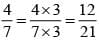QUESTION: 3

### What is the value of x if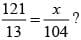Solution: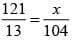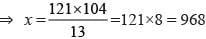QUESTION: 4

What result will be obtained when the sum of 65/12 and 8/3 is divided by their difference?

Solution: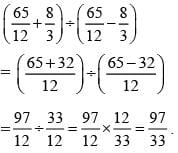QUESTION: 5

​What is multiplicative inverse of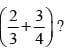Solution: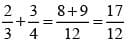Multiplicative inverse of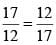QUESTION: 6

The cost of 15 articles is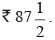What is the cost of one article?

Solution: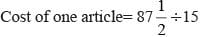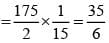QUESTION: 7

A bus is moving at an average speed of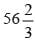km/hour. How much distance it cover in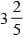hour?

Solution: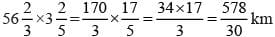QUESTION: 8

What should be added to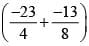to get1?

Solution: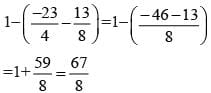QUESTION: 9

How many pieces each of length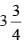m can be cut from a rope of length 60 m?

Solution: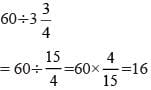QUESTION: 10

By what rational number should –8/65is multiplied to obtain 5/39?

Solution: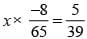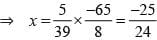Use Code STAYHOME200 and get INR 200 additional OFF Use Coupon Code